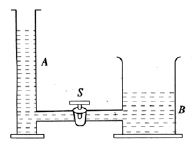# Similarity between Potential and Temperature and free Surface of Liquid

The role which is played by temperature and the height of free surface of liquid in heat and hydrostatics respectively, potential plays the same role in electrostatics. We know, if we connect two bodies thermally, there may be exchange of heat between them. The flow of heat does not depend on the mass of i.e. inherent heat within it but on the temperature. If we connect a highly heated body with another body which is much heater but of low in temperature, then heat will flow from the small body to the large body, though the amount of heat is much greater in the larger body than the smaller one.Two tubes A and B are placed at same horizontal level. They are connected by a tube with a stop cock S (Fig). Closing the stop cock water is poured into A and B tubes in such a way so the height of the water column is same in two tubes. As the diameter of B is much greater than that of A, to raise the water level at same height much more water is requires for tube B. Now if the stop cock is opened there would be no change in water height i.e. there is no flow of water. Though the amount of water is different in two tubes, as there is height is same, so there is no flow of water. Now if closing the stop cock a little amount water is poured into A tube, the amount of water in it will still be less than that of B, but the height of water level will increase slightly. After if the stop cock is opened, then water will flow from A to and the height of the water column will w same in both A and B. It is thus understood that flow of water does not depend on the amount of water rather the height.

Suppose two conductors are positively charged. The amount of charge in first conductor is greater than that of the second conductor but the potential of the first one is less than that of the second. Now if two conductors are connected electricity then positively charge will flow from second conductor to the first conductor. Though the amount of charge is greater in first conductor yet it will take charge because its potential is low. As a result of flow of charges when the potential of the two conductor become equal then the flow will stop.

Therefore it can be said, the role of temperature in heat, role of free surface of liquid in hydrostatic and the role of potential in electrostatic are same.